# How to determine the transfer function of an circuit of two parts?

#### cxf208

Joined May 30, 2022
1
Hello everyone, the circuit I am designing is like this: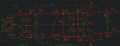I want to divide the circuit into different sections, and I want to know the transfer function of each section. I also want to know the total TF of the circuit.
Here is my question:
Can I calculate each TF of the different sections and multiply them together to get the whole TF?
What makes me confused is the circuit below.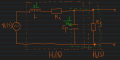I can calculate H1(s). I think it should be: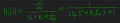I can also calculate the total TF H(s):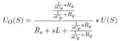->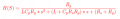If I want to keep H1(s) and H2(s) shown in the picture, I do not know how to get the H(s) by H1(s)&H2(s).
I must misunderstand something. Would anyone please give me some instructions?
Thank you very much.